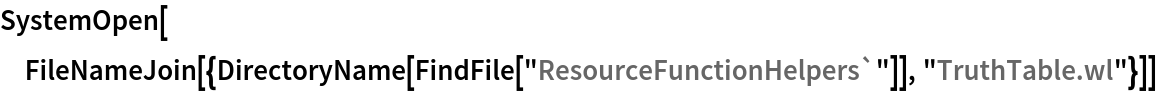#Function Repository Resource:

# TruthTable

Generate a truth table for a Boolean expression

Contributed by: Wolfram|Alpha Math Team (Jeremy Stratton-Smith)
 ResourceFunction["TruthTable"][expr,vars] creates a truth table for the logical expression expr with respect to vars. ResourceFunction["TruthTable"][boole] creates a truth table for the BooleanFunction boole with generated parameters. ResourceFunction["TruthTable"][…,format] creates a truth table with output form specified by format.

## Details

The input expr is expected to be composed of symbols and logical operators such as And, Or, Xor, Nor, etc. or else a List of such expressions.
The input vars is expected to be a symbol or list of symbols.
The input boole should be any BooleanFunction, and the output will generate a number of unused parameters equal to the value of BooleanVariables[boole], in addition to formatting the BooleanFunction as the simplest explicit form found using BooleanConvert.
The input format can be either "GridForm" (default) or "ListForm". If "ListForm" is entered, ResourceFunction["TruthTable"] will return an unformatted list of lists corresponding to the rows of the truth table.

## Examples

### Basic Examples (2)

Produce a truth table for a simple logical expression:

 In:=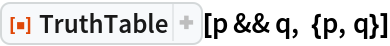Out=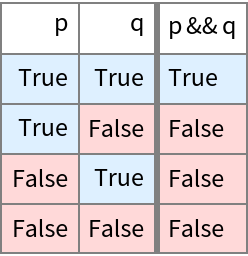You can also build up larger expressions:

 In:=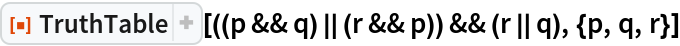Out=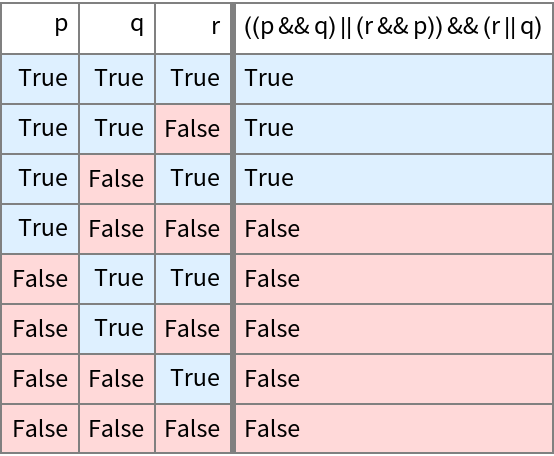### Scope (2)

TruthTable also accepts raw BooleanFunction inputs and produces an explicit logical expression for the function:

 In:=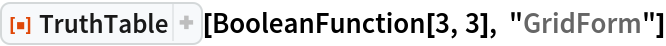Out=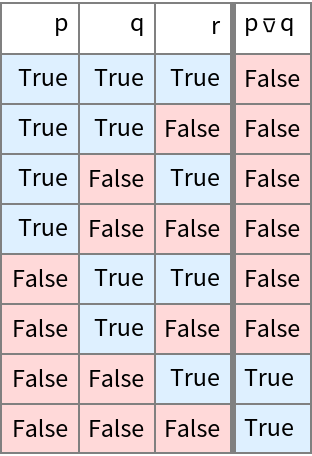Display the same result using the list format:

 In:=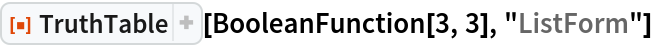Out=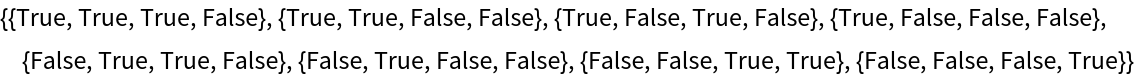### Applications (2)

Show DeMorgan's law by comparing two logical expressions:

 In:=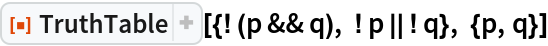Out=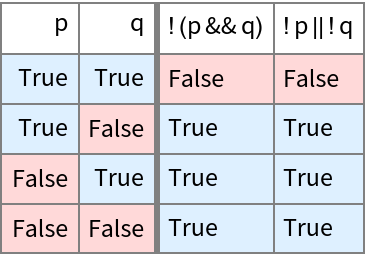Show that Implies is not associative by comparing the truth tables of two expressions:

 In:=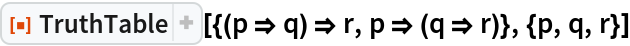Out=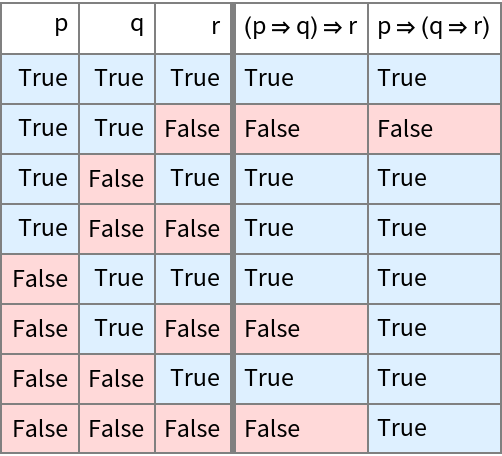### Neat Examples (1)

Use a truth table to easily determine if multiple logical expressions are equivalent:

 In:=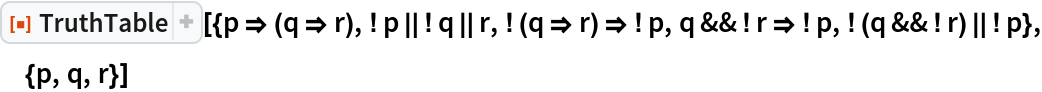Out=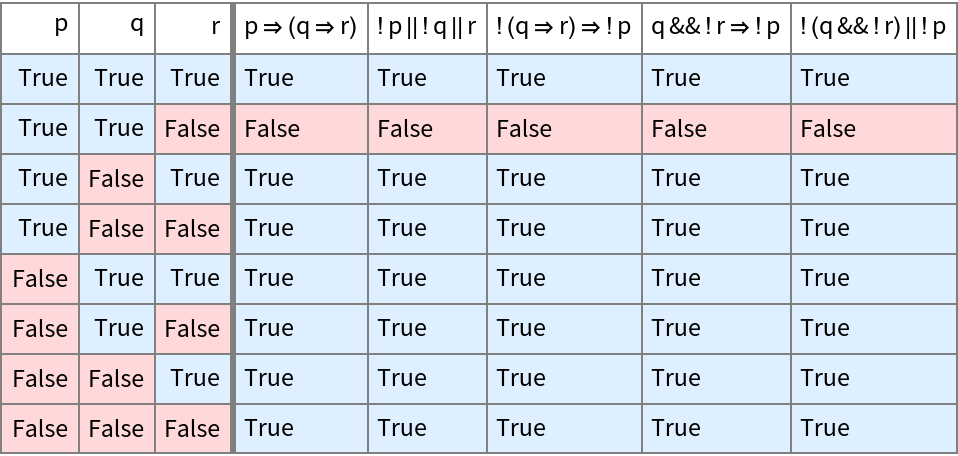## Author Notes

To view the full source code for TruthTable, evaluate the following:

 In:=